# Mensuration Class 6 Maths Notes - Chapter 10

Mensuration is a branch of mathematics which is a topic in geometry. It is a study of various geometrical shapes, their length, breadth, volume, and area for 2D as well as 3D shapes. Some important terminologies included in this topic are covered below.

## Introduction to Mensuration

### Mensuration

• Mensuration is the branch of mathematics that deals with the measurement of length, area or volume of various geometric shapes.

### Shapes

• A shape is the form of an object.
• Examples of two-dimensional shapes are square, rectangle and triangle, and of three-dimensional shapes are cube, cuboid and sphere.To know more about Mensuration, visit here.

## Perimeter

• Perimeter is the total length or total distance covered along the boundary of a closed shape.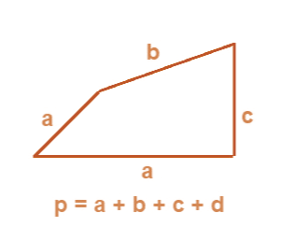• Perimeter of a circle is also called as the circumference of the circle.

### Perimeter of a Triangle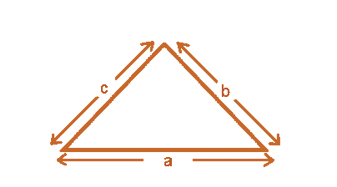• Perimeter of triangle = Sum of lengths of all sides = a + b + c.
• If the given triangle is equilateral that is if all the sides are equal (a = b = c),
then its perimeter is equal to 3 × length of one side of the triangle.

### Perimeter of a Rectangle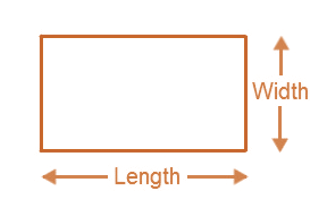• Perimeter of the rectangle = length ( l ) + length ( l ) + width (w) + width (w)

= 2× [length ( l ) + width (w)]

### Perimeter of a Square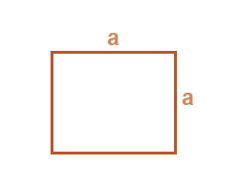Perimeter of square = 4 × length of a side = 4a

### Perimeter of an ‘n’ Sided Polygon

• A polygon is a closed shape made up of line segments.
• Perimeter of n sided polygon = n× length of one side.
• Example: Length of each side of a hexagon is a cm, then:
Perimeter of the hexagon = 6a cm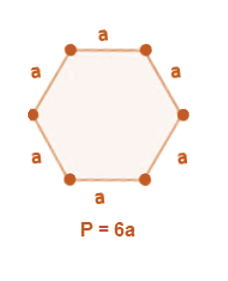### Perimeter of Irregular Sshapes

• Irregular shapes are the shapes which do not have all sides and angles equal.
• The perimeter of irregular shapes is equal to total length covered by the shape.
In the figure given below, perimeter is the sum of all sides.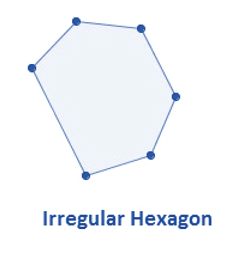## Area

• Area is the total amount of surface enclosed by a closed figure.

### Area of Square

• Area of a square = Side × Side =Side2=a2, where a is the length of each side.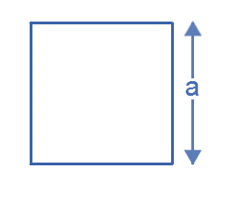### Area of Rectangle

•  Area = length ( l ) × breadth ( b)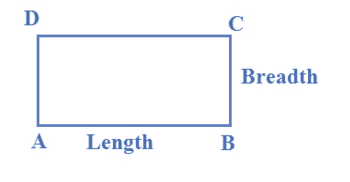### Area of Triangle

Area of triangle = (1/2) × base × height = (1/2) × b × h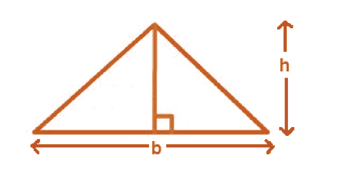To know Mensuration Formulas, visit here.

### Areas of different types of triangles

• Consider an acute and an obtuse triangle.
Area of each triangle = (1/2) × base × height = (1/2) × b × h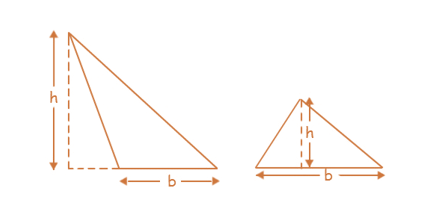### Visualisation of Area

• In the given graph, if the area of each small square is 1 cm2, then
Area of rectangle = l×b = 5×2 = 10cm2
Area of square = a×a = 2×2 =4cm2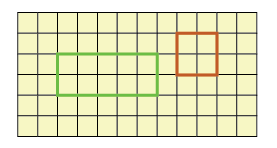#### For more information on Visualisation of Areas, watch the below video### Area of Irregular Shapes

• Area of an irregular figure can be calculated :
Step 1: Divide the irregular shape into regular shapes that you can recognize (eg. triangles, rectangles, circles and squares)
Step 2: Find the area of these individual shapes and add them. Sum will be the area of the irregular figure.
• Example: Area of the given figure = Area of MNCB + Area of AMGH + Area of EFND
= [ 5 × 9 + 4 × 2 + 3 × 3 ] cm2
= [45 + 8 + 9 ] cm2
=62 cm2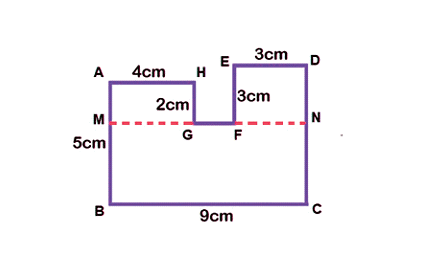To solve questions on Mensuration, visit here.

 Rectangle Triangles

## Frequently Asked Questions on CBSE Class 6 Maths Notes Chapter 10 Mensuration

Q1

### How can ‘perimeter’ be defined?

A perimeter is a closed path that encompasses, surrounds or outlines either a two dimensional shape or a one-dimensional length.

Q2

### What is an irregular hexagon?

An irregular hexagon is defined as a 6-sided polygon that is not regular-meaning, that all of the sides and angles do not have the same measure.

Q3

### What is a polygon?

A polygon is a closed shape with least three and usually has five or more, angles and straight sides.